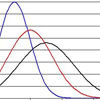#### You may also like### Over-booking

The probability that a passenger books a flight and does not turn up is 0.05. For an aeroplane with 400 seats how many tickets can be sold so that only 1% of flights are over-booked?### Into the Normal Distribution

Investigate the normal distribution### Normal Intersection

Explore the intersection possibilities for normal pdfs.

# Aim High

##### Age 16 to 18Challenge Level

For the second part you will need to use the formula for the expected value of a random variable $X$:
$$E(X) = \int^\infty_{-\infty} xf(x) dx$$

Don't forget that you can split an integral into two parts
$$\int^\infty_{-\infty} xf(x) dx=\int^a_{-\infty} xf(x) dx+\int^\infty_a xf(x) dx$$

You might also want to use the Erf function in Excel to work out the numbers and to change variables in any integrals to reduce them to standard $N(0,1)$ cases.